Courses

# NCERT Exemplar: Electrochemistry- 2 Notes | EduRev

## JEE : NCERT Exemplar: Electrochemistry- 2 Notes | EduRev

The document NCERT Exemplar: Electrochemistry- 2 Notes | EduRev is a part of the JEE Course Chemistry for JEE.
All you need of JEE at this link: JEE

Q.28. Can absolute electrode potential of an electrode be measured?
Ans. No, only the difference in potential between two electrodes can be measured. This is due to the reason that oxidation or reduction cannot occur alone. So, when we measure electrode potential of any electrode we have to take a reference electrode.

Q.29. Can E°Cell V or ∆rG° for cell reaction ever be equal to zero?
Ans. No, otherwise the reaction becomes non-feasible.
The reaction is feasible only at E°cell = positive or ∆rG° = negative.
When E° = ∆rG° = 0 the reaction reaches at equilibrium.

Q.30. Under what condition is E°Cell = 0 or ∆rG° = 0?
Ans. At the stage of chemical equilibrium in the cell we get E°cell = 0.
cell = 0
rG° = -n F E°cell
= −n x F x 0 = 0

Q.31. What does the negative sign in the expression E°Zn2+/Zn = − 0.76 V mean?
Ans. Greater the negative reactivity of standard reduction potential of metal greater is its reactivity. It means that Zn is more reactive than hydrogen. When zinc electrode will be connected to standard hydrogen electrode, Zn will get oxidised and H+ will get reduced.
Thus, zinc electrode will be the anode of the cell and hydrogen electrode will be the cathode of the cell.

Q.32. Aqueous copper sulphate solution and aqueous silver nitrate solution are electrolysed by 1 ampere current for 10 minutes in separate electrolytic cells. Will the mass of copper and silver deposited on the cathode be same or different? Explain your answer.
Ans. Different masses of Cu and Ag will be deposited at cathode. According to Faraday's second law of electrolysis amount of different substances liberated by same quantity of electricity passes through electrolyte solution is directly proportional to their chemical equivalent weight.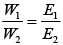Where, E1 and E2 have different values depending upon number of electrons required to reduce the metal ion. Thus, masses of Cu and Ag deposited will be different.

Q.33. Depict the galvanic cell in which the cell reaction is Cu + 2Ag+ → 2Ag + Cu2+
Ans. In a galvanic cell, oxidation half reaction is written on left hand side and reduction half reaction is on right hand side. Salt bridge is represented by parallel lines Cu | Cu2+ ||Ag+ | Ag.

Q.34. Value of standard electrode potential for the oxidation of Cl ions is more positive than that of water, even then in the electrolysis of aqueous sodium chloride, why is Cl oxidised at anode instead of water?
Ans. Under the condition of electrolysis of aqueous sodium chloride, oxidation of water at anode requires over potential. So, CI is oxidized at anode instead of water.
Possible oxidation half cell reactions occurring at anode are:
Cl(aq)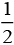Cl2 (g)+ e- ;   E°cell = 1.36V
2H2 O(l) O(g) 4H+ (aq) 4e- ; E°cell = 1.23V
Species having lower E°cell cell undergo oxidation first than the higher value but oxidation of H2O to O2 is kinetically so slow that it needs some overpotential.

Q.35. What is electrode potential?
Ans. The potential difference between the electrode and the electrolyte in an electrochemical cell is called electrode potential.

Q.36. Consider the following diagram in which an electrochemical cell is coupled to an electrolytic cell. What will be the polarity of electrodes ‘A’ and ‘B’ in the electrolytic cell?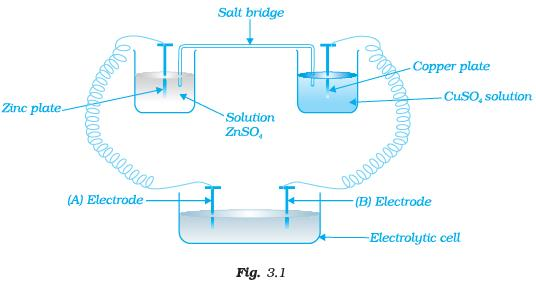Ans. The cell drawn above represents electrochemical cell in which two different electrodes are fitted in their respective electrolytic solutions and cell drawn at bottom represents electrolytic cell. Cell representation can be represented as Zn | Zn2+ || Cu2+|Cu.
Zn is losing electrons which are going towards electrode (A) and copper is accepting electron from electrode B. Hence,
Charge on electrode A = positive (+)
Charge on electrode B = negative (−)

Q.37. Why is alternating current used for measuring resistance of an electrolytic solution?
Ans. Alternating current is used in electrolysis so that concentration of ions in the solution remains constant and exact value of resistance is measured.

Q.38. A galvanic cell has electrical potential of 1.1V. If an opposing potential of 1.1V is applied to this cell, what will happen to the cell reaction and current flowing through the cell?

Ans. When an opposing potential of 1.1V is applied to a galvanic cell having electrical potential of 1.1V then cell reaction stops completely and no current will flow through the cell.

Q.39. How will the pH of brine (aq. NaCl solution) be affected when it is electrolysed?

Ans. The pH of the solution will rise as NaOH is formed in the electrolytic cell.
Chemical reactions occurring at cell when aqueous brine solution is electrolysed are as follows: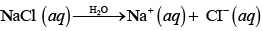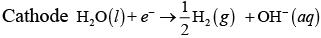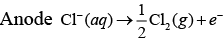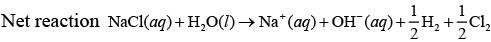Q.40. Unlike dry cell, the mercury cell has a constant cell potential throughout its useful life. Why?
Ans. Life time of any cell depends upon ions present in cell. Ions are not involved in the overall cell reaction of mercury cell. Hence, mercury cell has a constant cell potential throughout its useful life.

Q.41. Solutions of two electrolytes ‘A’ and ‘B’ are diluted. The Λm of ‘B’ increases 1.5 times while that of A increases 25 times. Which of the two is a strong electrolyte? Justify your answer.

Ans. Strong electrolytes dissociate almost completely even on high concentration. Therefore, concentration of such solutions remains almost same on dilution. Electrolyte 'B' is stronger than 'A' because in 'B' the number of ions remains the same on dilution, but only interionic attraction decreases.
Therefore, ∧m increases only 1.5 times, while in case of weak electrolyte on dilution, number of constituent ions increases.

Q.42. When acidulated water (dil.H2SO4 solution) is electrolysed, will the pH of the solution be affected? Justify your answer.
Ans. Since pH of solution depends upon concentration of H+present in solutions, pH of the solution will not be affected as [H+] remains constant.
At anode 2H2O → O2+ 4H+e-
At cathode 4H+ + 4e → 2H2

Q.43. In an aqueous solution how does specific conductivity of electrolytes change with addition of water?
Ans. Conductivity of solution due to total ions present in per unit volume of solution is known as specific conductivity. Specific conductivity decreases due to decrease in the number of ions per unit volume. We add water to aqueous solution, number of ions present in per unit volume decreases.

Q.44. Which reference electrode is used to measure the electrode potential of other electrodes?
Ans. Standard hydrogen electrode (SHE) is the reference electrode whose electrode potential is taken to be zero. The electrode potential of other electrodes are measured with respect to it.

Q.45. Consider a cell given below
Cu|Cu2+|| Cl-|Cl2,Pt
Write the reactions that occur at anode and cathode
Ans. Cell reaction represented in the question is composed of two half cell reactions. These reactions are as follows
At anode Cu → Cu2+ + 2e
At cathode Cl2 + 2e → 2Cl
Copper is getting oxidised at anode and Cl2 is getting reduced at cathode.

Q.46. Write the Nernst equation for the cell reaction in the Daniel cell. How will the ECell be affected when concentration of Zn2+ ions is increased?
Ans. Zn + Cu2 → Zn2+ Cu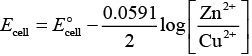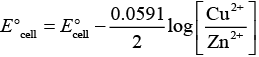According to this equation
cell is directly dependent on concentration of Cu2+ and inversely dependent upon concentration of Zn2+ ions.
Ecell decreases when concentration of Zn2+ ions is increased.

Q.47. What advantage do the fuel cells have over primary and secondary batteries?
Ans. Primary batteries contain a limited amount of reactants and are discharged when the reactants have been consumed. Secondary batteries can be recharged but it takes a long time. Fuel cell runs continuously as long as the reactants are supplied to it and products are removed continuously.

Q.48. Write the cell reaction of a lead storage battery when it is discharged. How does the density of the electrolyte change when the battery is discharged?
Ans. When a lead storage battery is discharged then the following cell reaction takes place
Pb + PbO2 + 2H2SO4 → 2PbSO4 + 2H2O
Density of electrolyte depends upon number of constituent ions present in per unit volume of electrolyte solution in this case density of electrolyte decreases as water is formed and sulphuric acid is consumed as the product during discharge of the battery.

Q.49. Why on dilution the Λm of CH3COOH increases drastically, while that of CH3COONa increases gradually?
Ans. In the case of CH3COOH, which is a weak electrolyte, the number of ions increases on dilution due to an increase in degree of dissociation.
CH3COOH + H2O ⇌ CH3COO+ H3O+
In case of strong electrolyte, the number of ions remains the same but the interionic attraction decreases.

MATCHING TYPE

Note : Match the items of Column I and Column II in the following questions.
Q.50. Match the terms given in Column I with the units given in Column II.

 Column I Column II (i) ∧m (a) S cm–1 (ii) ECell (b) m-1 (iii) κ (c) S cm2 mol–1 (iv) G* = l/a (d) V

Ans. (i) → c, (ii) → d, (iii) → a, (iv)→ b
Solution.

 Column I Column II(Unit of parameter) (i) ∧m (c) S cm2 mol–1 (ii) ECell (d) V (iii) κ(conductivity) (a) S cm–1 (iv) G* = l/a (b) m-1

Q.51. Match the terms given in Column I with the items given in Column II.

 Column I Column II (i) Λm (a) Intensive property (ii) EºCell (b) Depends on number of ions/volume (iii) κ (c) Extensive property (iv) ∆rGCell (d) Increases with dilution

Ans. (i) → d, (ii) → a, (iii)→ b, (iv) → c
Solution.

(i) Λm (molar conductivity) is the conductivity due to number of ions furnished by one mole of electrolyte. As dilution increases number of ions present in the solution also increases hence molar conductivity increases.
(ii)Cell of any atom/ion does not depend upon number of atoms/ions. Hence EºCell of any atom/ion is an intensive properties.
(iii) κ represents specific conductivity which depends upon number of ions present in per unit volume.
(iv)rGCell is an extensive property as it depends upon number of particles (species)

Q.52. Match the items of Column I and Column II.

 Column I Column II (i) Lead storage battery (a) maximum efficiency (ii) Mercury cell (b) prevented by galvanisation (iii) Fuel cell (c) gives steady potential (iv) Rusting (d) Pb is anode, PbO2 is cathode

Ans. (i)→ d, (ii) → c, (iii)→ a, (iv)→ b
Solution.

(i) Chemical reaction occurring on lead storage battery can be represented as
At anode Pb(s) + SO42−(aq) → PbSO4 (s) + 2e
At cathode Pb(s) + SO42−(aq)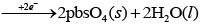Thus, Pb is anode and PbO2 is cathode.
(ii) Mercury cell does not include ions during their function hence produce steady current.
(iii) Fuel cell has maximum efficiency as they produce energy due to combustion reaction of fuel.
(iv) Rusting is prevented by corrosion.

Q.53. Match the items of Column I and Column II.

 Column I Column II (i) κ (a) I × t (ii) Λm (b) Λm/Λ0m (iii) α (c) κ/c (iv) Q (d) G*/R

Ans. (i) → d, (ii) → c, (iii)→ b, (iv) → a
Solution.

(i) Conductivity (k) =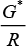(ii) Molar conductivity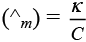(iii) Degree of dissociation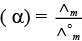(iv) Charge Q =I x t
where, Q is the quantity of charge in coulomb when I ampere of current is passed through an electrolyte for t second.

Q.54. Match the items of Column I and Column II.

 Column I Column II (i) Lechlanche cell (a) cell reaction 2H2 + O2 → 2H2O (ii) Ni–Cd cell (b) does not involve any ion in solution and is used in hearing aids. (iii) Fuel cell (c) rechargeable (iv) Mercury cell (d) reaction at anode, Zn → Zn2+ + 2e– (e) converts energy of combustion into electrical energy

Ans. (i)→ d, (ii) → c, (iii) → a, e (iv) → b
Solution.

(i) The electrode reaction occurring at Lechlanche cell are
At anode Zn(s) → Zn2 + 2e
At cathode MnO2NH4+ e → MnO (OH) NH3
(ii) Ni-Cd cell is rechargeable. So, it has more life time.
(iii) Fuel cell produces energy due to combustion. So, fuel cell converts energy of combustion into electrical energy e.g., 2H+ O2 → 2H2O
(iv) Mercury cell does not involve any ion in solution and is used in hearing aids.

Q.55. Match the items of Column I and Column II on the basis of data given below: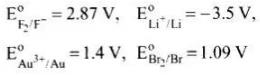Column I Column II (i) F2 (a) metal is the strongest reducing agent (ii) Li (b) metal ion which is the weakest oxidising agent (iii) Au3+ (c) non metal which is the best oxidising agent. (iv) Br– (d) unreactive metal (v) Au (e) anion that can be oxidised by Au3+ (vi) Li + (f) anion which is the weakest reducing agent (vii) F – (g) metal ion which is an oxidising agent

Ans. (i) → c, (ii)  → a, (iii) → g, (iv) → e, (v)  → d, (vi)  → b, (vii)  → f
Solution.

(i) F2 is a non-metal and best oxidising agent because SRP of F2 is + 2.87 V.
(ii) Li is a metal and strongest reducing agent because SRP of Li is −3.05 V.
(iii) Au3+ is a metal ion which is an oxidising agent as SRP of Au3+ is +1.40V.
(iv) Br is an anion that can be oxidised by
Au3+ as Au3+ (E° = 1.40) is greater than
Br (E° = 1,09V).
(v) Au is an unreactive metal.
(vi) Li+ is a metal ion having least value of SRP (− 3.05 V), hence it is the weakest oxidising agent.
(vii) F is an anion which is the weakest reducing agent as F /F2 has low oxidation potential (−2.87 V).

ASSERTION AND REASON TYPE

Note : In the following questions a statement of assertion followed by a statement of reason is given. Choose the correct answer out of the following choices.
(i) Both assertion and reason are true and the reason is the correct explanation of assertion.
(ii) Both assertion and reason are true and the reason is not the correct explanation of assertion.
(iii) Assertion is true but the reason is false.
(iv) Both assertion and reason are false.
(v) Assertion is false but reason is true.

Q.56. Assertion : Cu is less reactive than hydrogen.
Reason :Cu2+/Cu is negative.
(i) Both assertion and reason are true and the reason is the correct explanation of assertion.
(ii) Both assertion and reason are true and the reason is not the correct explanation of assertion.
(iii) Assertion is true but the reason is false.
(iv) Both assertion and reason are false.
(v) Assertion is false but reason is true.
Ans. (iii)
Solution.

Electrode potential of Cu2+ /Cu is + 0.34V and Electrode potential of 2H+ /H2 is 0.00 V.
Hence, correct reason is due to positive value of Cu2+ /Cu it looses electron to H+ and get reduces, while H2 gas evolves out.

Q.57. Assertion : ECell should have a positive value for the cell to function.
Reason : Ecathode < Eanode
(i) Both assertion and reason are true and the reason is the correct explanation of assertion.
(ii) Both assertion and reason are true and the reason is not the correct explanation of assertion.
(iii) Assertion is true but the reason is false.
(iv) Both assertion and reason are false.
(v) Assertion is false but reason is true.
Ans. (iii)
Solution.

Feasibility of chemical reaction depends on Gibbs free energy which is related to E°cell 3S
ΔG- = -nFE- cell
When value of E°cell is positive then ΔG° becomes negative. Hence, reaction becomes feasible, Correct reason is Ecathode > Eanode.

Q.58. Assertion : Conductivity of all electrolytes decreases on dilution.
Reason : On dilution number of ions per unit volume decreases.
(i) Both assertion and reason are true and the reason is the correct explanation of assertion.
(ii) Both assertion and reason are true and the reason is not the correct explanation of assertion.
(iii) Assertion is true but the reason is false.
(iv) Both assertion and reason are false.
(v) Assertion is false but reason is true.
Ans. (i)
Solution.

Since, conductivity depends upon number of ions per unit volume. Therefore, the conductivity of all electrolytes decreases on dilution due to decrease in number of ions per unit volume.

Q.59. Assertion : Λm for weak electrolytes shows a sharp increase when the electrolytic solution is diluted.
Reason : For weak electrolytes degree of dissociation increases with dilution of solution.
(i) Both assertion and reason are true and the reason is the correct explanation of assertion.
(ii) Both assertion and reason are true and the reason is not the correct explanation of assertion.
(iii) Assertion is true but the reason is false.
(iv) Both assertion and reason are false.
(v) Assertion is false but reason is true.
Ans. (i)
Solution.

Molar conductivity of weak electrolytic solution increases on dilution, because as we add excess water to increase the dilution degree of dissociation increases which leads to increase in number of ions in the solution. Thus, Λm show a very sharp increase.

Q.60. Assertion : Mercury cell does not give steady potential.
Reason : In the cell reaction, ions are not involved in solution.
(i) Both assertion and reason are true and the reason is the correct explanation of assertion.
(ii) Both assertion and reason are true and the reason is not the correct explanation of assertion.
(iii) Assertion is true but the reason is false.
(iv) Both assertion and reason are false.
(v) Assertion is false but reason is true.
Ans. (v)
Solution.

Assertion is false but reason is true, correct assertion is mercury cell gives steady potential.
Reason is correct as ions are not involved in cell reaction.

Q.61. Assertion : Electrolysis of NaCl solution gives chlorine at anode instead of O2.
Reason : Formation of oxygen at anode requires overvoltage.
(i) Both assertion and reason are true and the reason is the correct explanation of assertion.
(ii) Both assertion and reason are true and the reason is not the correct explanation of assertion.
(iii) Assertion is true but the reason is false.
(iv) Both assertion and reason are false.
(v) Assertion is false but reason is true.
Ans. (i)
Solution.

Electrolysis of NaCl is represented by following chemical reactions At cathode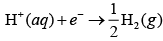At anode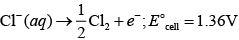2H2O(aq) O2(g) 4H+ (aq) 4e-; E°Cell 1.23V
E°cell for this reaction has lower value but formation of oxygen at anode requires over potential.

Q.62. Assertion : For measuring resistance of an ionic solution an AC source is used.
Reason : Concentration of ionic solution will change if DC source is used.
(i) Both assertion and reason are true and the reason is the correct explanation of assertion.
(ii) Both assertion and reason are true and the reason is not the correct explanation of assertion.
(iii) Assertion is true but the reason is false.
(iv) Both assertion and reason are false.
(v) Assertion is false but reason is true.
Ans. (i)
Solution.

Concentration of ionic solution changes on using DC current as a source of energy while on passing AC current concentration does not change. Hence, AC source is used for measuring resistance.

Q.63. Assertion : Current stops flowing when ECell = 0.
Reason : Equilibrium of the cell reaction is attained.
(i) Both assertion and reason are true and the reason is the correct explanation of assertion.
(ii) Both assertion and reason are true and the reason is not the correct explanation of assertion.
(iii) Assertion is true but the reason is false.
(iv) Both assertion and reason are false.
(v) Assertion is false but reason is true.
Ans. (i)
Solution.

Current stop flowing when Ecell = 0
As at Ecell = 0 reaction reaches the equilibrium.

Q.64. Assertion : EAg+/Ag increases with increase in concentration of Ag+ ions.
Reason : EAg+/Ag E has a positive value.
(i) Both assertion and reason are true and the reason is the correct explanation of assertion.
(ii) Both assertion and reason are true and the reason is not the correct explanation of assertion.
(iii) Assertion is true but the reason is false.
(iv) Both assertion and reason are false.
(v) Assertion is false but reason is true.
Ans. (ii)
Solution.

Both assertion and reason are correct but reason is not the correct explanation of assertion.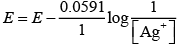E = E° + 0.059 log {Ag+]
Thus E Ag+/Ag increases with increase in concentration of Ag+

Q.65. Assertion : Copper sulphate can be stored in zinc vessel.
Reason : Zinc is less reactive than copper.
(i) Both assertion and reason are true and the reason is the correct explanation of assertion.
(ii) Both assertion and reason are true and the reason is not the correct explanation of assertion.
(iii) Assertion is true but the reason is false.
(iv) Both assertion and reason are false.
(v) Assertion is false but reason is true.
Ans. (iv)
Solution.

Copper sulphate can't be stored in zinc vessel as zinc is more reactive than copper due to negative value of standard reduction potential of Zn.

Q.66. Consider the Fig. 3.2 and answer the following questions.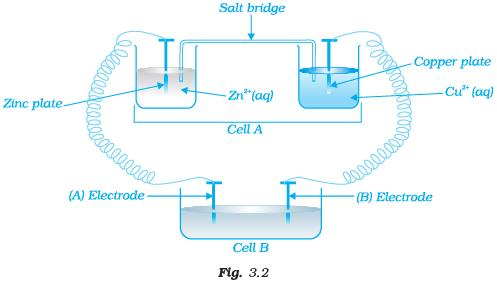(i) Cell ‘A’ has ECell = 2V and Cell ‘B’ has ECell = 1.1V which of the two cells ‘A’ or ‘B’ will act as an electrolytic cell. Which electrode reactions will occur in this cell?
(ii) If cell ‘A’ has ECell = 0.5V and cell ‘B’ has ECell = 1.1V then what will be the reactions at anode and cathode?

Ans. (i) Cell 'B' will act as electrolytic cell due to its lesser value of emf.
The electrode reactions will be
At cathode Zn2+ + 2e→ Zn
At anode Cu → Cu2+ + 2e
(ii) If cell 'B' has higher emf, it acts as galvanic cell.
Now it will push electrons into cell ‘A’
In this case, the reactions will be
Zn →Zn2+ + 2e (At anode)
Cu2+ +2e → Cu (At cathode)

Q.67. Consider Fig. 3.2 and answer the questions (i) to (vi) given below.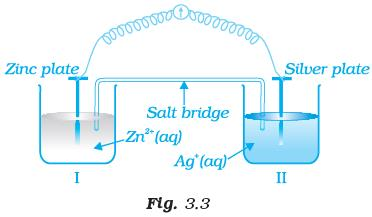(i) Redraw the diagram to show the direction of electron flow.
(ii) Is silver plate the anode or cathode?
(iii) What will happen if salt bridge is removed?
(iv) When will the cell stop functioning?
(v) How will concentration of Zn2+ ions and Ag+ ions be affected when the cell functions?
(vi) How will the concentration of Zn2+ ions and Ag+ ions be affected after the cell becomes ‘dead’?
Ans. (i) Electrons move from Zn to Ag as E' is more negative for Zn, so Zn undergoes oxidation and Ag+ undergoes reduction.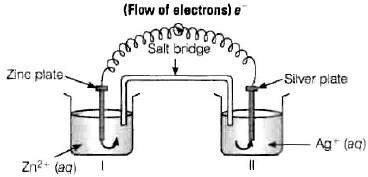(ii) Ag is the cathode as it is the site of reduction where Ag+ takes electrons from medium and deposit at cathode.
(iii) Cell will stop functioning because cell potential drops to zero. At E = 0 reaction reaches equilibrium.
(iv) When Ecell = 0 because at this condition reaction reaches to equilibrium.
(v) Concentration of Zn2+ ions will increase and concentration of Ag+ ions will decrease because Zn is converted into Zn2+ and Ag+ is converted into Ag.
(vi) When Ecell = 0 equilibrium is reached and concentration of Zn2+ ions and Agwill not change.

Q.68. What is the relationship between Gibbs free energy of the cell reaction in a galvanic cell and the emf of the cell? When will the maximum work be obtained from a galvanic cell?
Ans. If concentration of all reacting species is unity, then Ecell = E°cell and ΔG° = -nFE°cell where, ΔrG° is standard Gibbs energy of the reaction
cell = emf of the cell
nF = charge passed
If we want to obtain maximum work from a galvanic cell then charge has to be passed reversibly.
The reversibly work done by a galvanic cell is equal to decrease in its Gibbs energy.
ΔrG = -nFEcell
As Ecell is an intensive parameter but ΔrG is an extensive thermodynamic property and the value depends on n.
For reaction, Zn (s) + Cu2+ (aq) → Zn2+ (aq)+ Cu (s)in a galvanic cell.
ΔrG = -2FEcell [Here, n = 2]

Offer running on EduRev: Apply code STAYHOME200 to get INR 200 off on our premium plan EduRev Infinity!

## Chemistry for JEE

223 videos|446 docs|334 tests

,

,

,

,

,

,

,

,

,

,

,

,

,

,

,

,

,

,

,

,

,

;